# 数据挖掘入门系列教程（三点五）之决策树

## 数据挖掘入门系列教程（三点五）之决策树

### 决策树（Decision Tree）介绍

Decision Tree是一类较为常见的机器学习的方法。它既可以作为分类算法，也可以作为回归算法。

• Q：性别要求？
• A：不是女的不要。
• Q：年龄要求？
• A：大于我3岁的不要
• Q：专业要求？
• A：非CS不要
• ……• 根节点：包含样本的全集
• 内部节点：对应特征属性测试
• 叶节点：代表决策的结果

### 结点的选择

“信息熵”（information entropy）：可以度量样本集合中的“纯度”。 在信息世界，熵越高，表示蕴含越多的信息，熵越低，则信息越少。 而根节点需要包含所以的样本，则`根结点的熵最大`

#### 信息熵（Information Entropy）

[ Ent(D) = – sum_{k=1}^{n}p_klog_2p_k\ Ent(D)越大，则D的纯度越小，也就是说集合D越混乱。 ]

#### 信息增益（information gain）

[ Gain(D,a) = Ent(D) – sum_{v=1}^Vfrac{|D^v|}{|D|}Ent(D^v) ]

### 决策树生成算法流程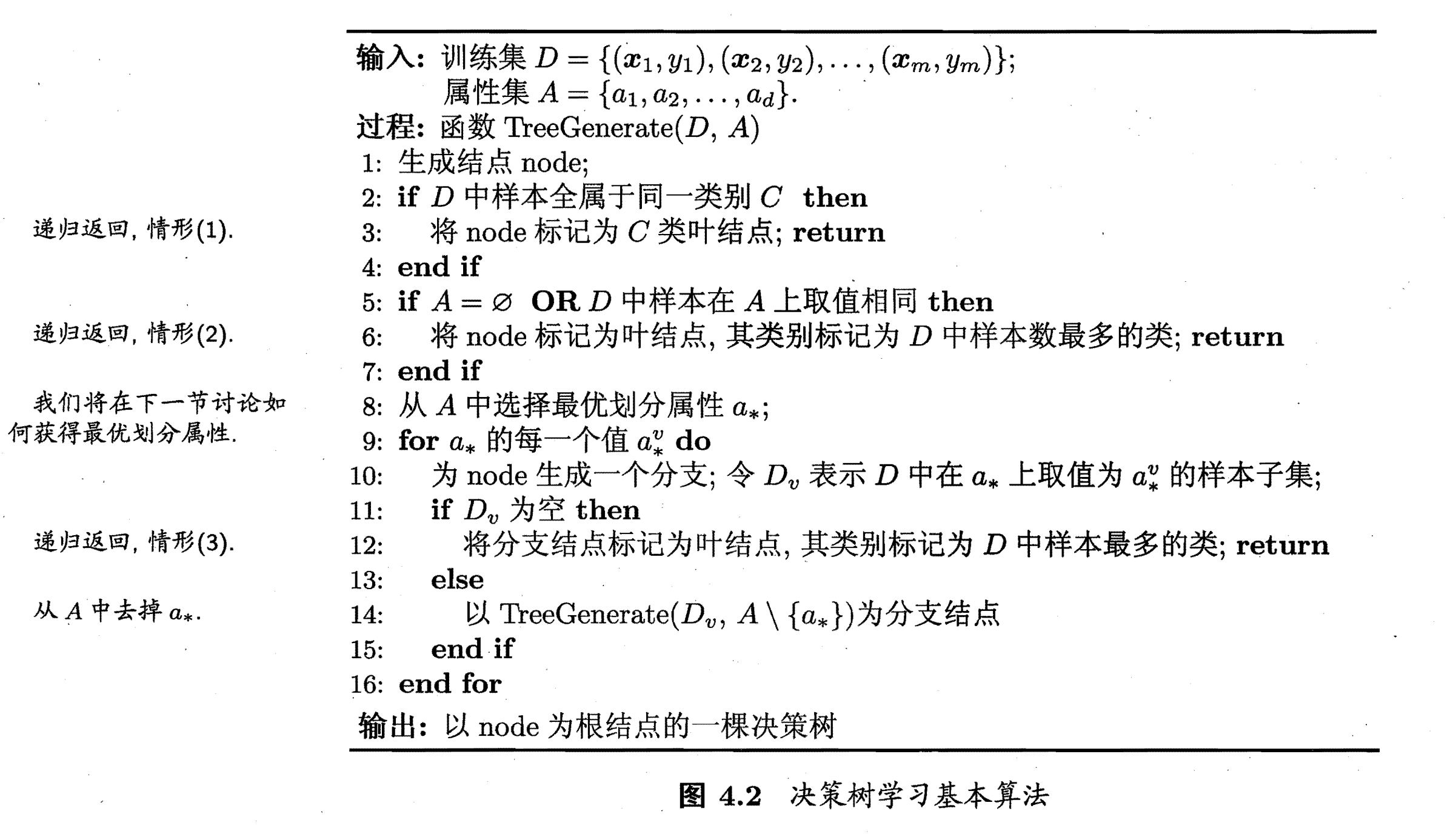• 当前结点包含的样本全属于同一类别，无需划分
• 当前属性集为空，或是所有样本在所有属性上取值相同，无法划分
• 当前结点包含的样本集合为空，不能划分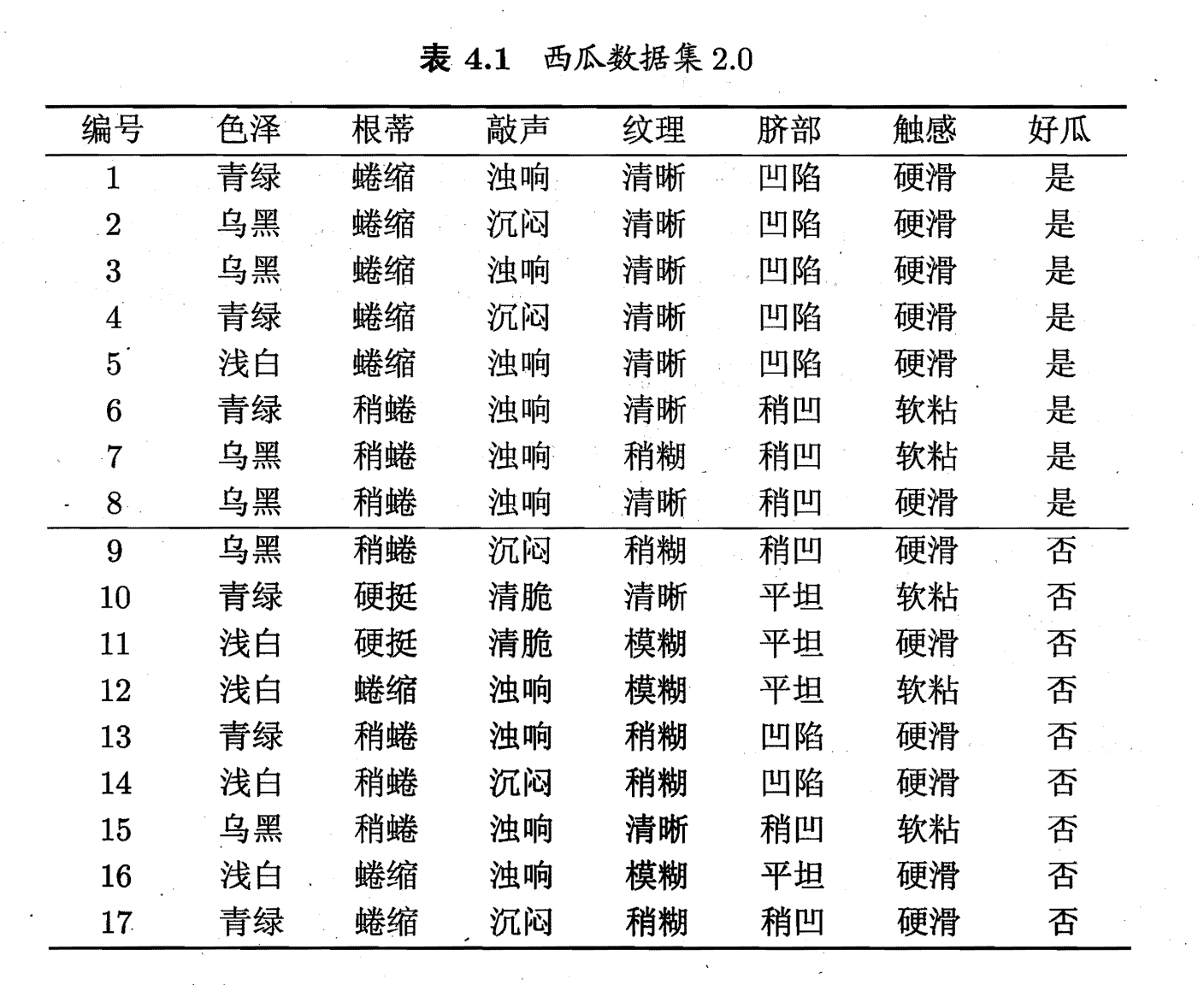[ Ent(D) = -sum_{k=1}^2p_klog_2p_k = -(frac{8}{17}log_2frac{8}{17} + frac{9}{17}log_2frac{9}{17}) = 0.998 ]

[ D^1(色泽=青绿) = {1,4,6,10,13,17} \ 在D^1中p_1 = frac{3}{6}，p_2=frac{3}{6}\ D^2(色泽=乌黑) = {2,3,7,8,9,15}\ 在D^2中p_1 = frac{4}{6}，p_2=frac{2}{6}\ D^3(色泽=浅白) = {5,11,12,14,16}\ 在D^2中p_1 = frac{1}{5}，p_2=frac{4}{5} \ 其中集合中的数字代表表格中的编号 ]

[ Ent(D^1)=-(frac{3}{6}log_2frac{3}{6}+frac{3}{6}log_2frac{3}{6}) = 1.00 \ Ent(D^2)=-(frac{4}{6}log_2frac{4}{6}+frac{2}{6}log_2frac{2}{6}) = 0.918 \ Ent(D^3)=-(frac{1}{5}log_2frac{1}{5}+frac{4}{5}log_2frac{4}{5}) = 0.722 \ ]

[ begin{equation} begin{aligned} Gain(D，色泽) &= Ent(D) – sum_{v=1}^3frac{|D^v|}{|D|}Ent(D^v)\ &= 0.99 – （frac{6}{17}times 1.00 + frac{6}{17}times0.918 +frac{5}{17}times0.722） \ &= 0.109 end{aligned} end{equation} ]

[ begin{equation} begin{split} & Gain(D，根蒂) = 0.143；Gain(D，敲声) = 0.141；\ & Gain(D，纹理) = 0.381；Gain(D，脐部) = 0.289； \ & Gain(D，触感) = 0.006；Gain(D，色泽)=0.109； end{split} end{equation} ]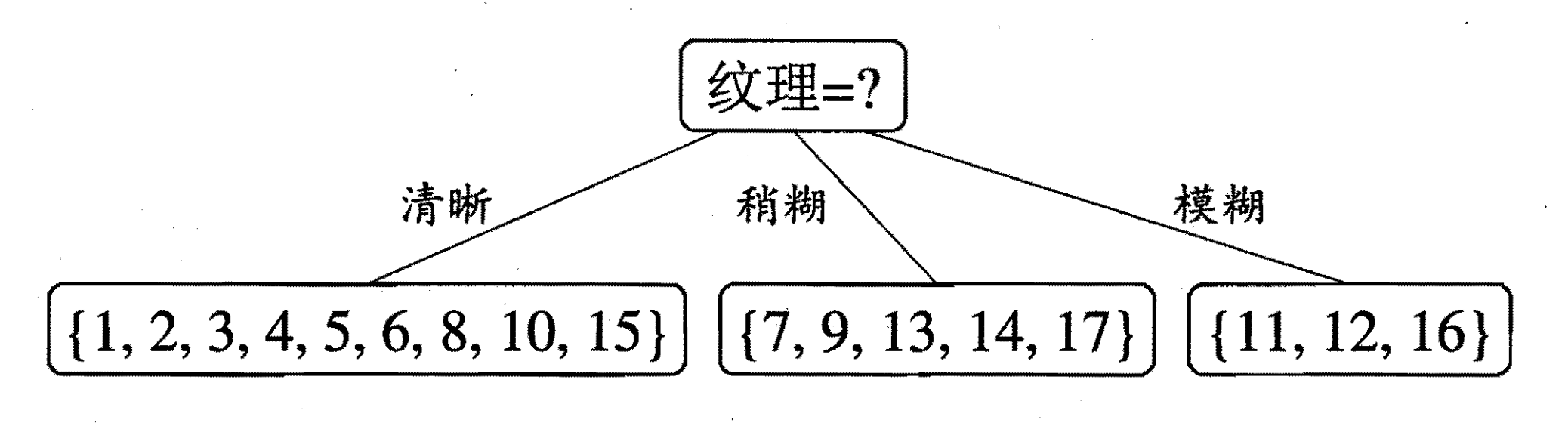[ begin{equation} begin{split} & Gain(D^1，色泽) = 0.043；Gain(D^1，根蒂) = 0.458；\ &Gain(D^1，敲声) = 0.331； Gain(D^1，触感) = 0.458；\ &Gain(D^1，脐部)=0.458； end{split} end{equation} ]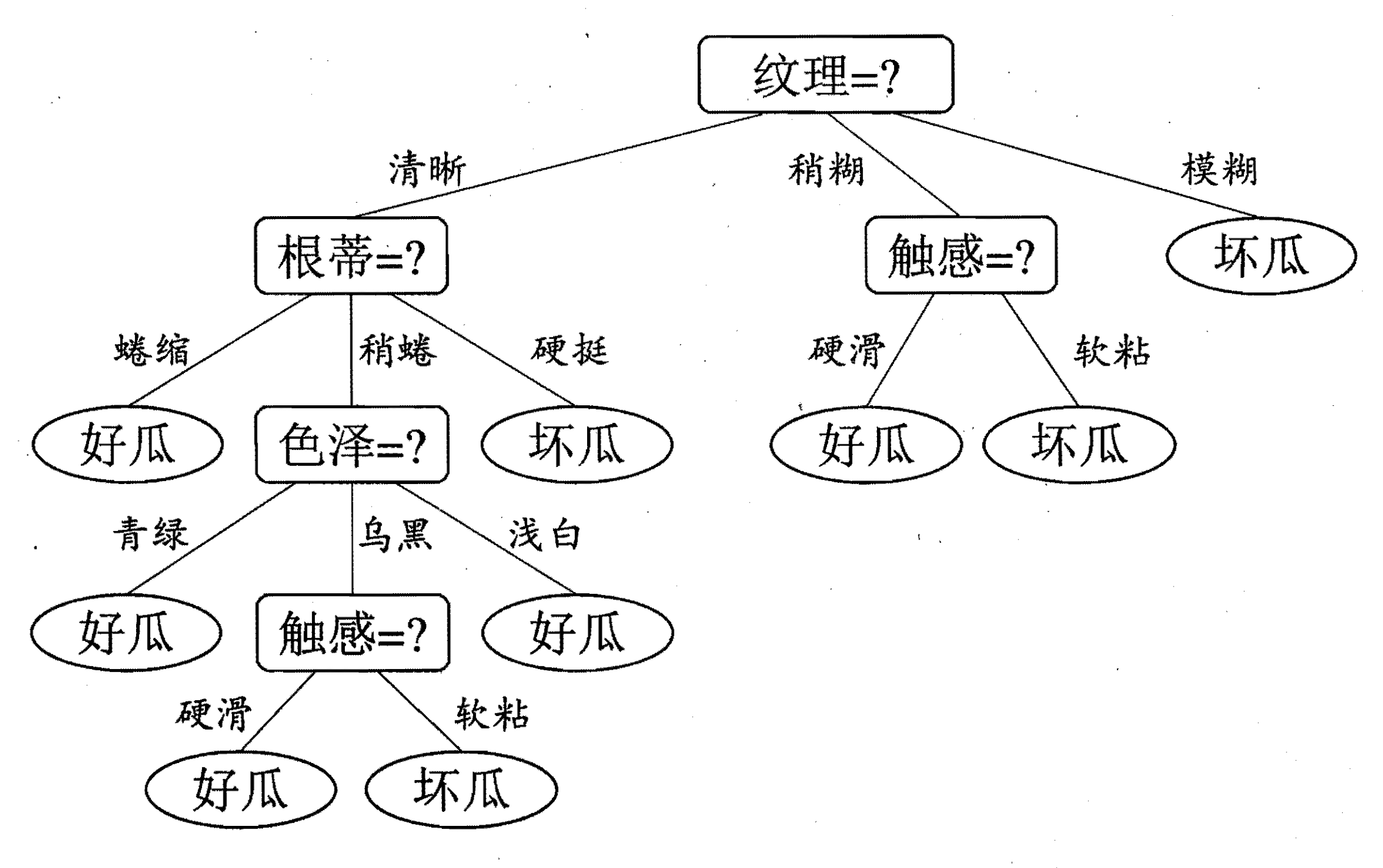### 增益率（Gain Ratio）

[ begin{equation} begin{split} &Gain_ratio(D,a) = frac{Gain(D,a)}{IV(a)} \ &其中\ &IV(a) = -sum_{v=1}^Vfrac{D^v}{D}log_2frac{D^v}{D} end{split} end{equation} ]

(IV(a))称之为属性(a)`固有值`(intrinsic value)，属性(a)的可能取值越多（划分的(D^v)的集合越多），(IV(a))就会越大。若像编号一样进行划分（每一个划分的集合中只有一个样本），随着编号的增多，(IV(a))的取值如下图：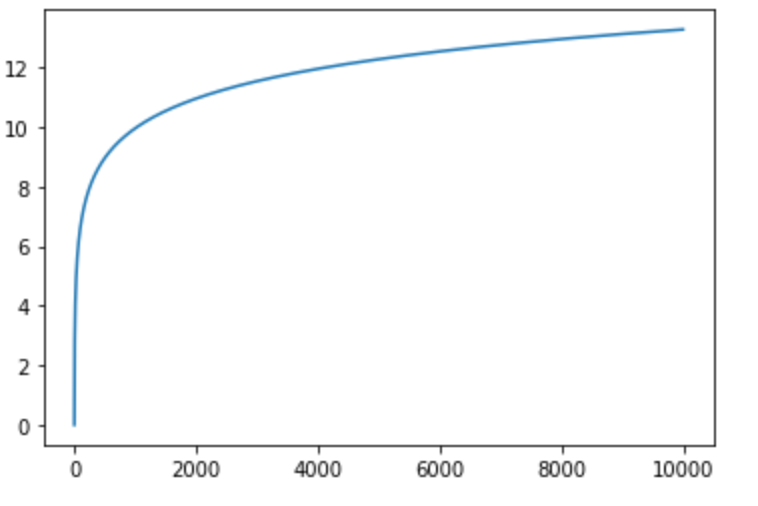### 基尼指数（Gini Index）

[ begin{equation} begin{aligned} Gini(D) &= sum_{k=1}^{|n|}sum_{k'neq k}p_kp_{k'}\ &=1 – sum_{k=1}^{|n|}p_k^2 end{aligned} end{equation} ]

(Gini(D)) 反映了从数据集中随机抽取两个样本，其类别标记不一致的概率。因此， \$Gini(D) \$越大，则数据集越复杂，纯度越低。

[ Gini_index(D,a) = sum_{v=1}^Vfrac{D^v}{D}Gini({D^v}) ]### 剪枝（pruning）处理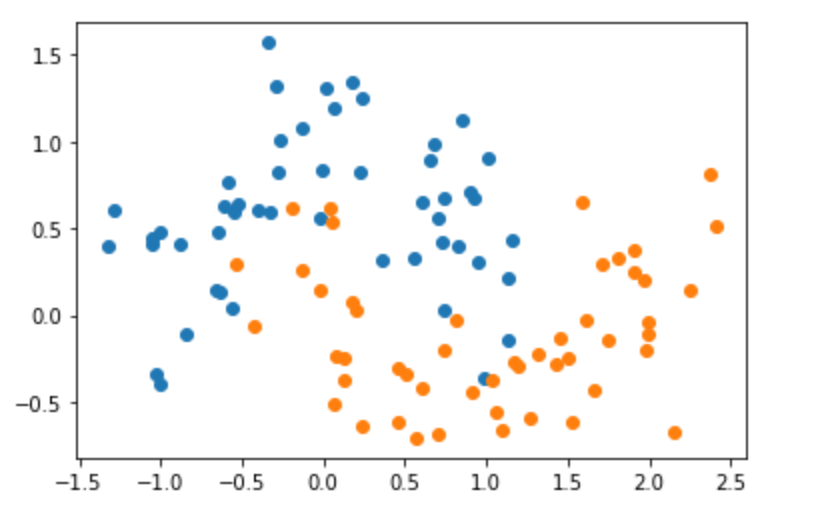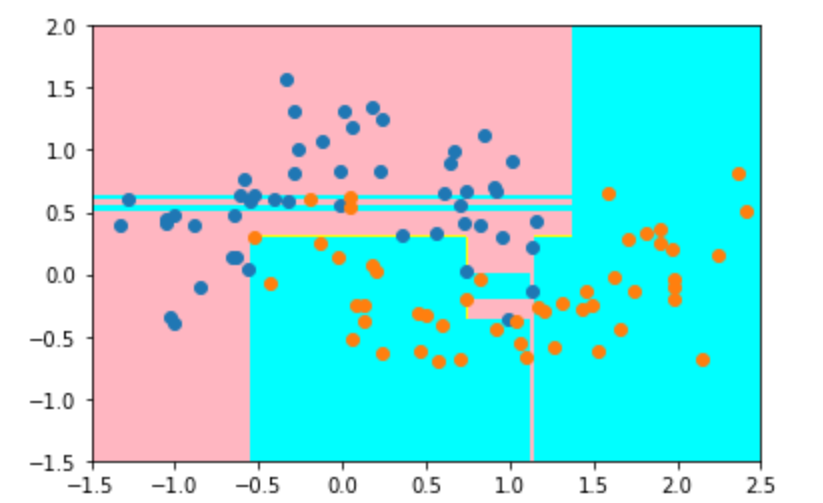• 预剪枝（prepruning）
• 后剪枝（post-pruning）

#### 预剪枝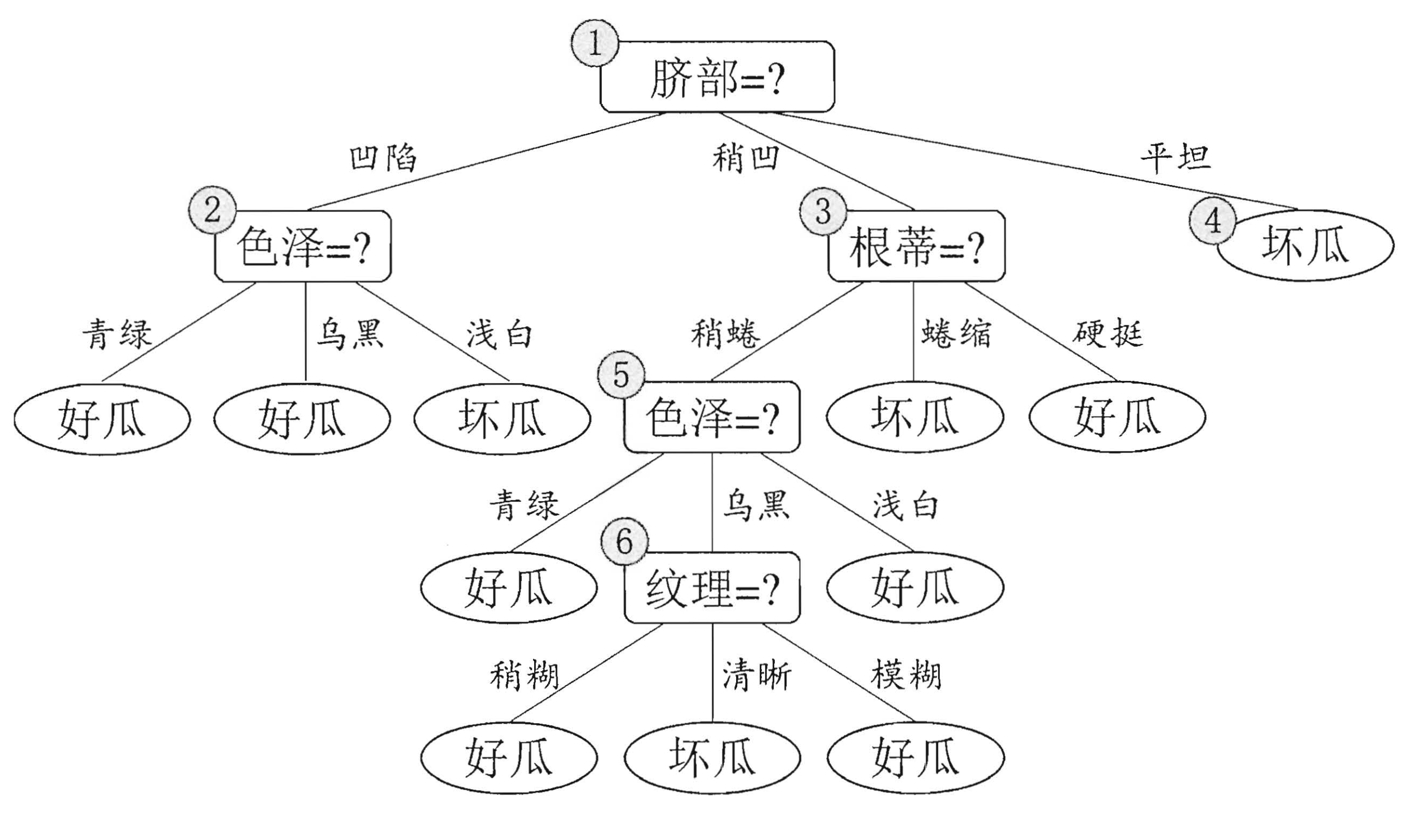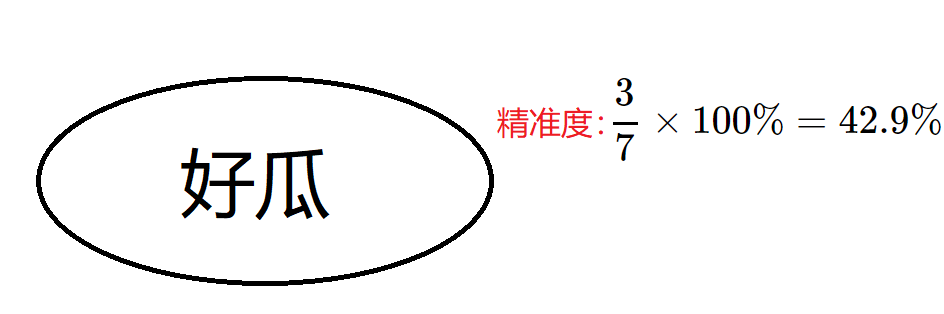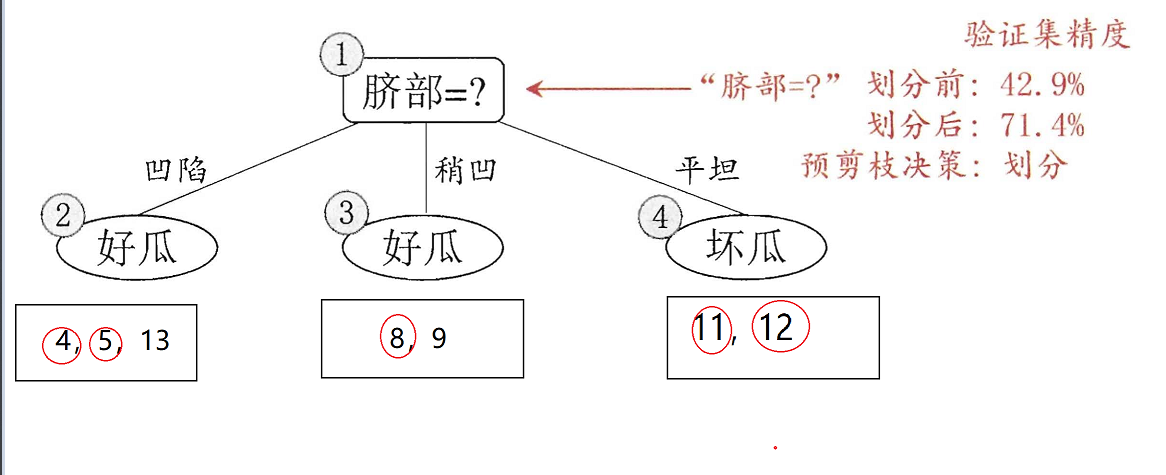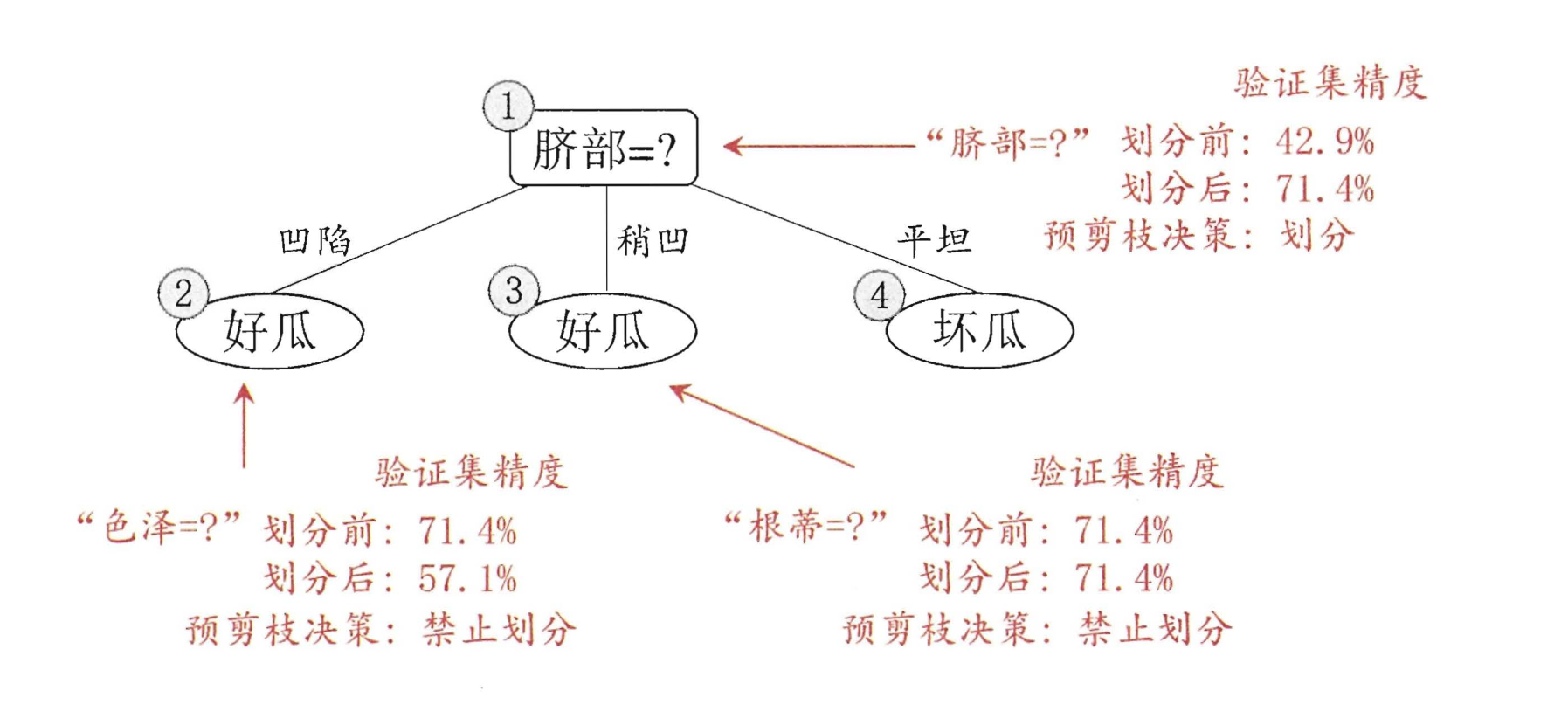#### 后剪枝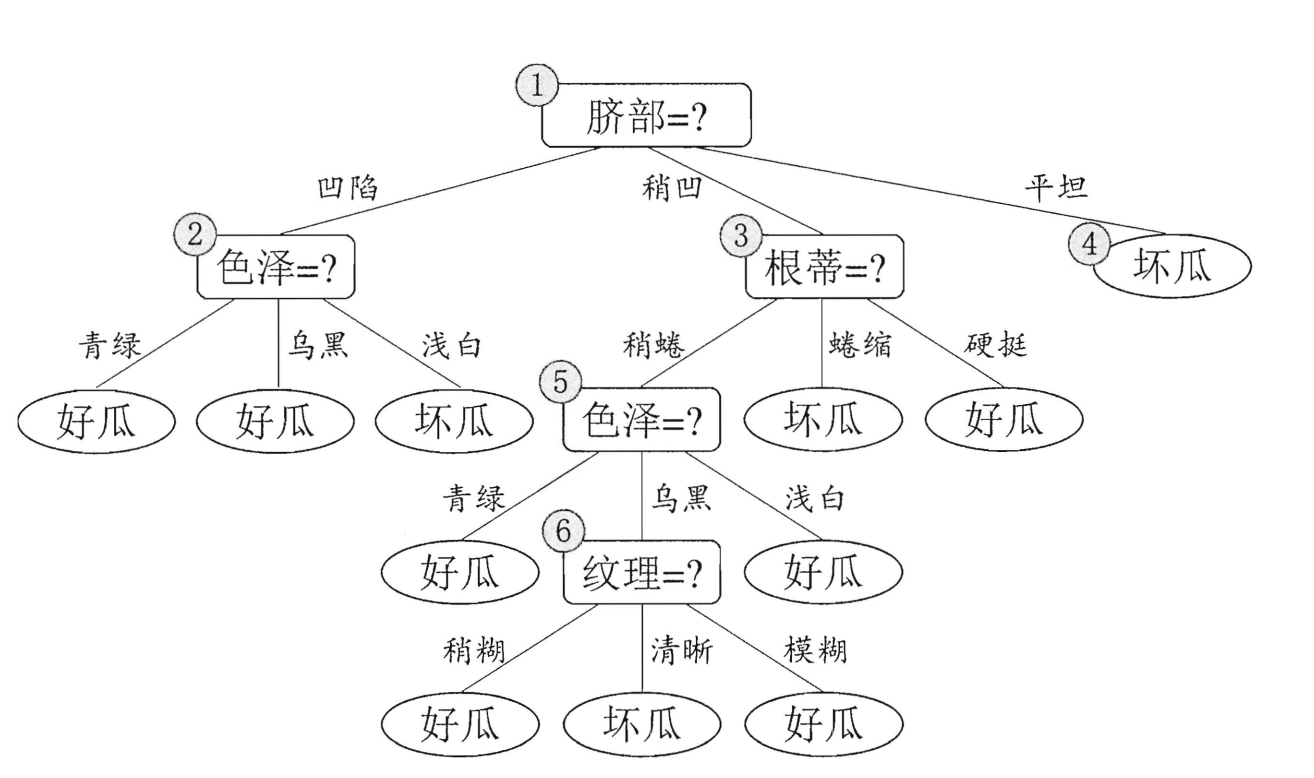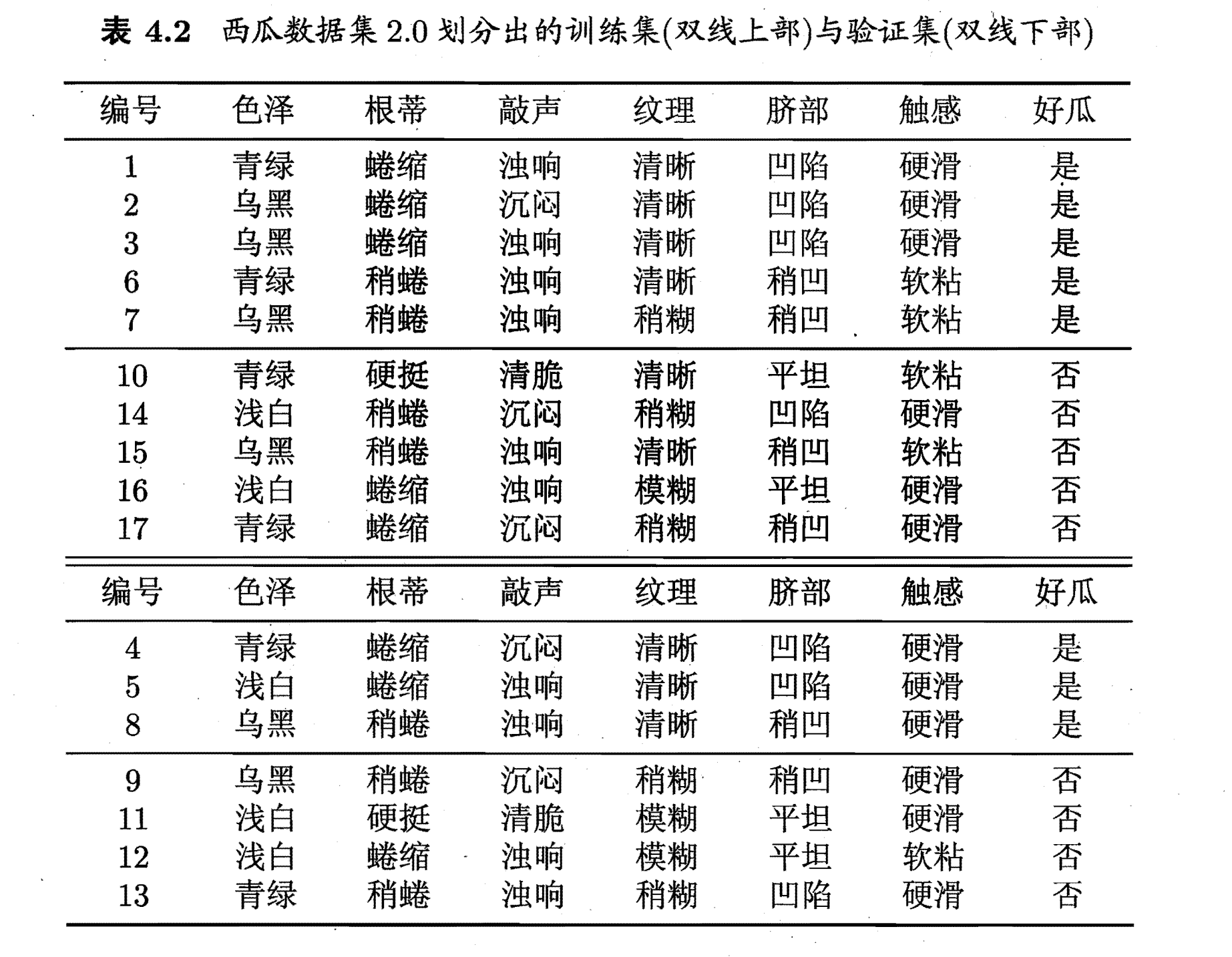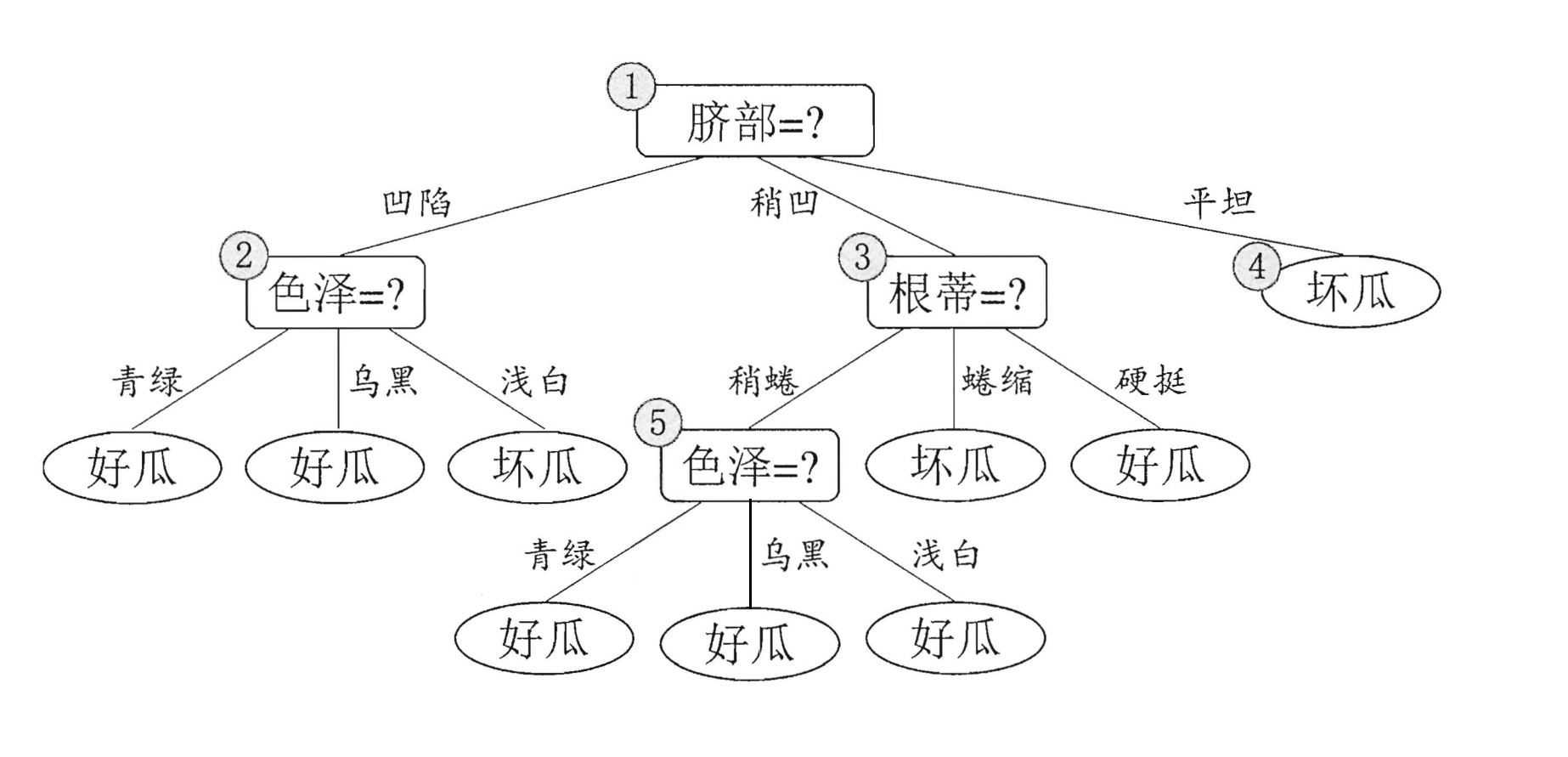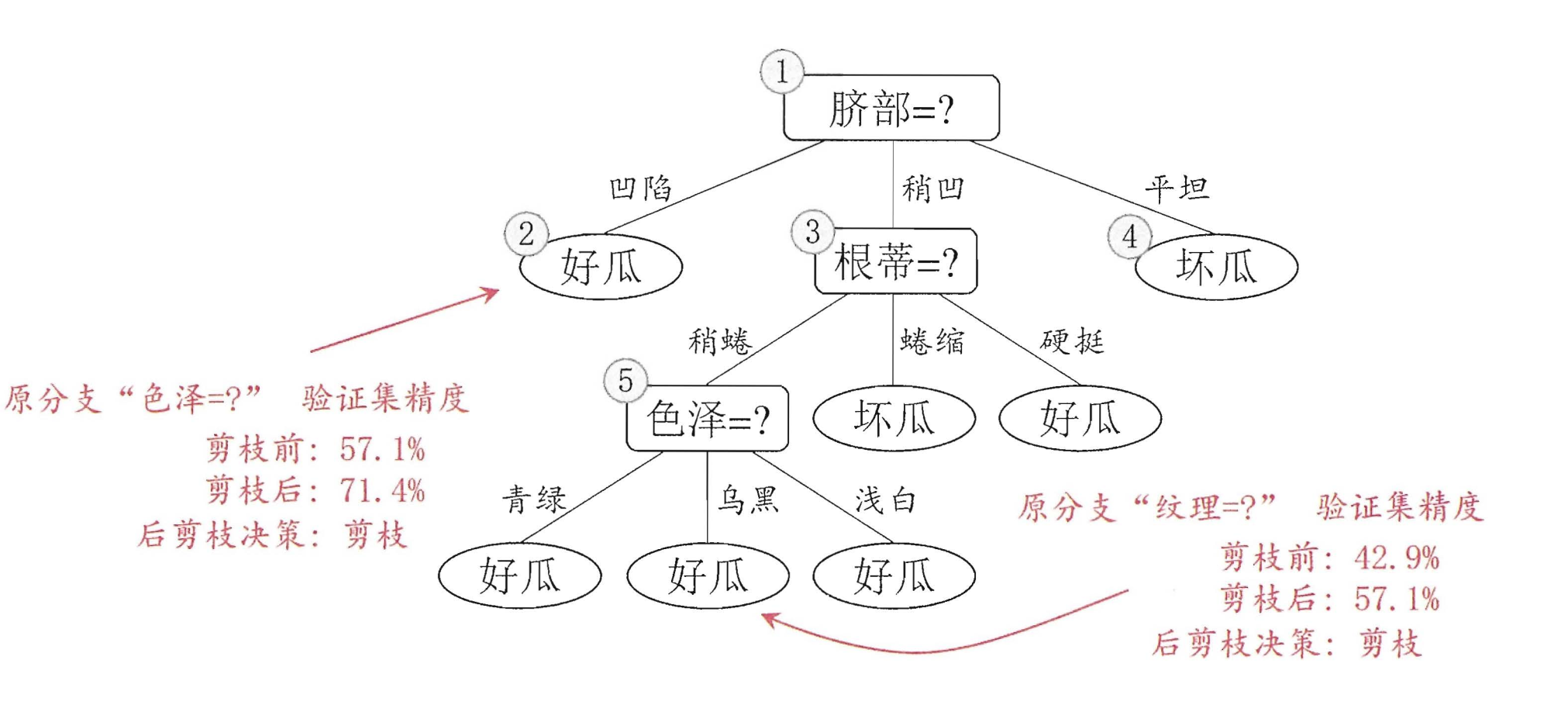### 随机森林（Random Forest | RF）

• 样本随机：Bagging算法
• 属性随机

#### 样本随机

ps：通过以上这种取样得到的集合(D_i)中间可能会有重复的元素（因为是有放回的抽取元素）

#### 属性随机

ps：在这种情况下，(m)个属性中是没有重复的属性的。

#### 随机森林的优缺点

1. 它可以出来很高维度（特征很多）的数据，并且不用降维，无需做特征选择
2. 它可以判断特征的重要程度
3. 可以判断出不同特征之间的相互影响
4. 不容易过拟合
5. 训练速度比较快，容易做成并行方法
6. 实现起来比较简单
7. 对于不平衡的数据集来说，它可以平衡误差。
8. 如果有很大一部分的特征遗失，仍可以维持准确度。

1. 随机森林已经被证明在某些噪音较大的分类或回归问题上会过拟合。
2. 对于有不同取值的属性的数据，取值划分较多的属性会对随机森林产生更大的影响，所以随机森林在这种数据上产出的属性权值是不可信的

### 参考

1. 《西瓜书》决策树——第四章
2. 维基百科：随机森林 Bagging算法